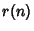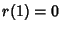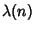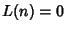## Pólya Conjecture

Letbe a Positive Integer andthe number of (not necessarily distinct) Prime Factors of(with). Letbe the number of Positive Integerswith an Odd number of Prime factors, andthe number of Positive Integerswith an Even number of Prime factors. Pólya conjectured thatis, whereis the Liouville Function.

The conjecture was made in 1919, and disproven by Haselgrove (1958) using a method due to Ingham (1942). Lehman (1960) found the first explicit counterexample,, and the smallest counterexamplewas found by Tanaka (1980). The firstfor whichare, 4, 6, 10, 16, 26, 40, 96, 586, 906150256, ... (Tanaka 1980, Sloane's A028488). It is unknown ifchanges sign infinitely often (Tanaka 1980).

References

Haselgrove, C. B. A Disproof of a Conjecture of Pólya.'' Mathematika 5, 141-145, 1958.

Ingham, A. E. On Two Conjectures in the Theory of Numbers.'' Amer. J. Math. 64, 313-319, 1942.

Lehman, R. S. On Liouville's Function.'' Math. Comput. 14, 311-320, 1960.

Sloane, N. J. A. Sequence A028488 in The On-Line Version of the Encyclopedia of Integer Sequences.'' http://www.research.att.com/~njas/sequences/eisonline.html.

Tanaka, M. A Numerical Investigation on Cumulative Sum of the Liouville Function'' [sic]. Tokyo J. Math. 3, 187-189, 1980.